# NCERT Solutions for Class 8 Maths Chapter 4 Exercise 4.3 – Practical Geometry

NCERT Solutions for Class 8 Maths Chapter 4 Exercise 4.3 – Practical Geometry, has been designed by the NCERT to test the knowledge of the student on the topic – Constructing a Quadrilateral – When two adjacent sides and three angles are known

### NCERT Solutions for Class 8 Maths Chapter 4 Exercise 4.3 – Practical Geometry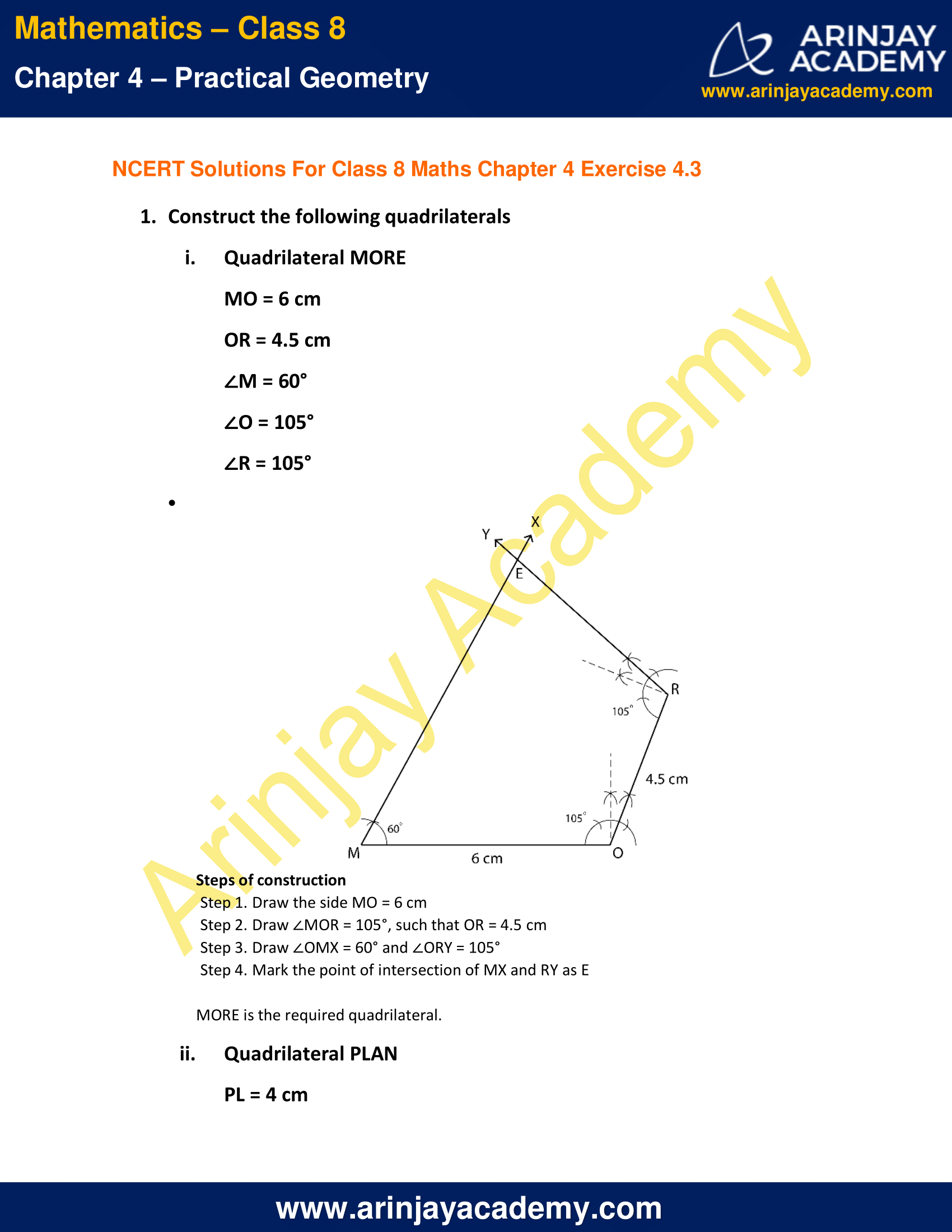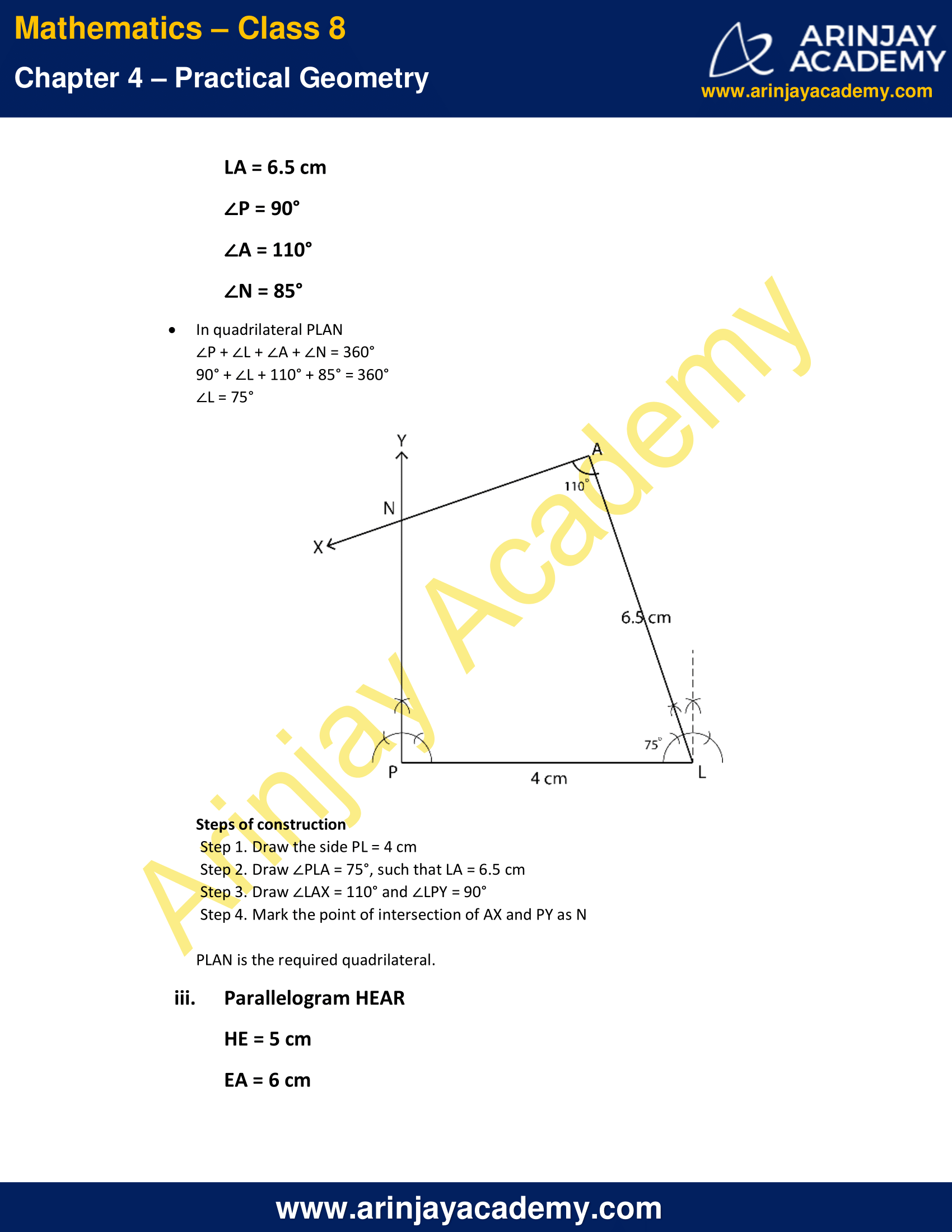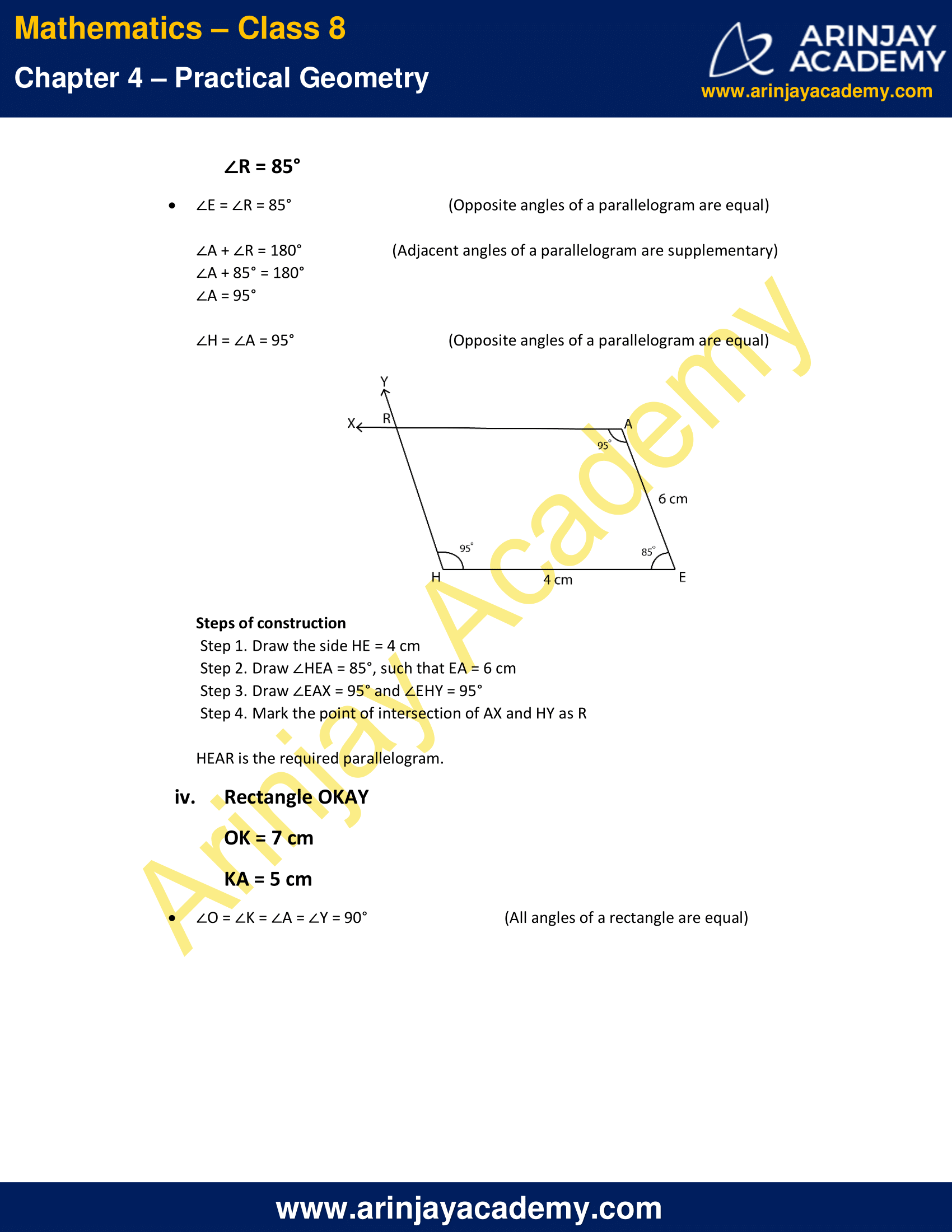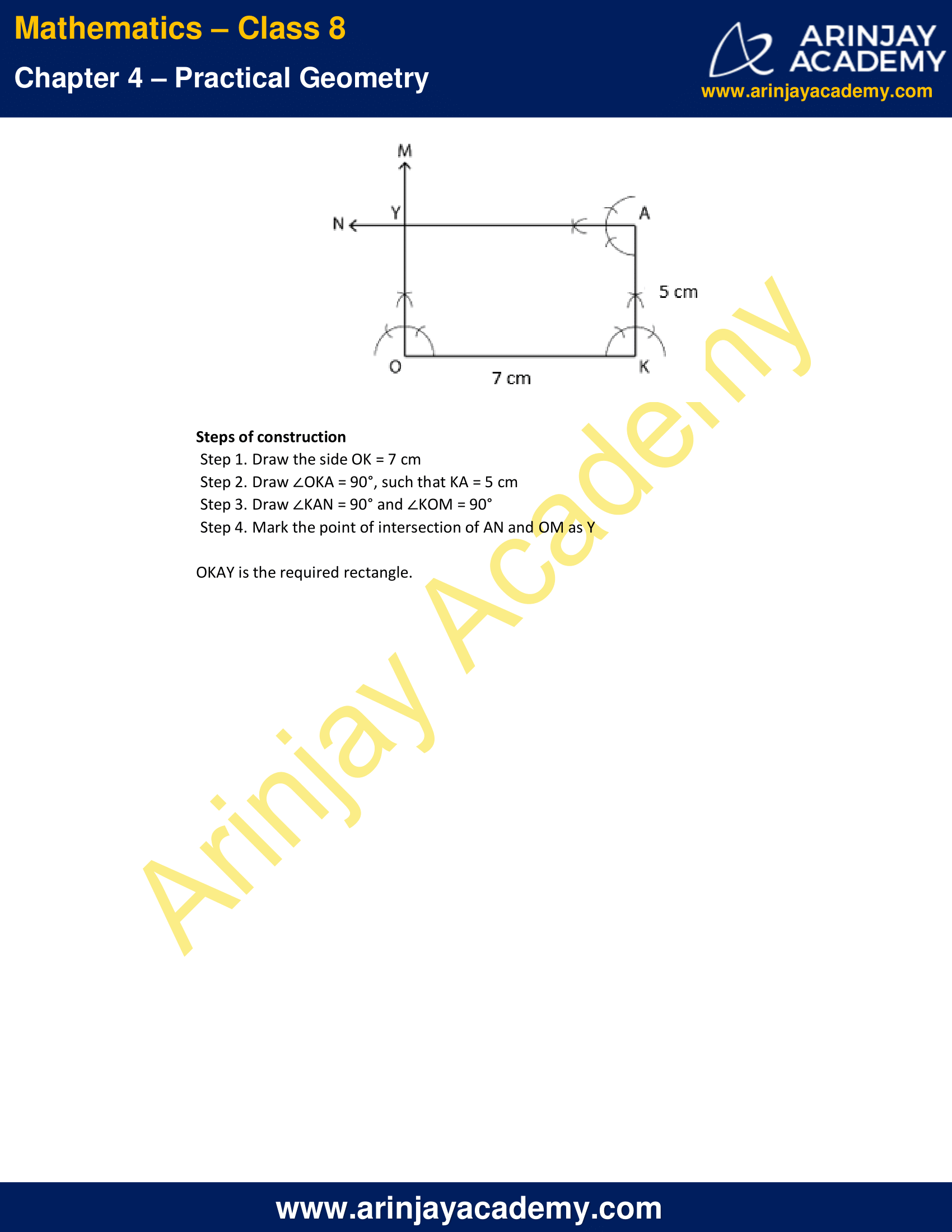NCERT Solutions for Class 8 Maths Chapter 4 Exercise 4.3 – Practical Geometry

MO = 6 cm
OR = 4.5 cm
∠M = 60°
∠O = 105°
∠R = 105°

Solution:Steps of construction

• Step 1. Draw the side MO = 6 cm
• Step 2. Draw ∠MOR = 105°, such that OR = 4.5 cm
• Step 3. Draw ∠OMX = 60° and ∠ORY = 105°
• Step 4. Mark the point of intersection of MX and RY as E

PL = 4 cm
LA = 6.5 cm
∠P = 90°
∠A = 110°
∠N = 85°

Solution:

∠P + ∠L + ∠A + ∠N = 360°
90° + ∠L + 110° + 85° = 360°
∠L = 75°Steps of construction

• Step 1. Draw the side PL = 4 cm
• Step 2. Draw ∠PLA = 75°, such that LA = 6.5 cm
• Step 3. Draw ∠LAX = 110° and ∠LPY = 90°
• Step 4. Mark the point of intersection of AX and PY as N

iii. Parallelogram HEAR

HE = 5 cm
EA = 6 cm
∠R = 85°

Solution:

∠E = ∠R = 85°   (Opposite angles of a parallelogram are equal)
∠A + ∠R = 180°  (Adjacent angles of a parallelogram are supplementary)
∠A + 85° = 180°
∠A = 95°
∠H = ∠A = 95°   (Opposite angles of a parallelogram are equal)Steps of construction

• Step 1. Draw the side HE = 4 cm
• Step 2. Draw ∠HEA = 85°, such that EA = 6 cm
• Step 3. Draw ∠EAX = 95° and ∠EHY = 95°
• Step 4. Mark the point of intersection of AX and HY as R

HEAR is the required parallelogram.

iv. Rectangle OKAY

OK = 7 cm
KA = 5 cm

Solution:

∠O = ∠K = ∠A = ∠Y = 90°  (All angles of a rectangle are equal)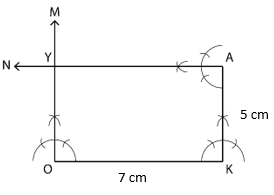Steps of construction

• Step 1. Draw the side OK = 7 cm
• Step 2. Draw ∠OKA = 90°, such that KA = 5 cm
• Step 3. Draw ∠KAN = 90° and ∠KOM = 90°
• Step 4. Mark the point of intersection of AN and OM as Y

OKAY is the required rectangle.

The next Exercise for NCERT Solutions for Class 8 Maths Chapter 4 Exercise 4.4 – Practical Geometry can be accessed by clicking here

NCERT Solutions for Class 8 Maths Chapter 4 Exercise 4.3 – Practical Geometry